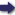(London :  Kegan Paul, Trench, Trübner & Co.,  1910.)

 Tools

## Search this bookPrev Page 315 Next``` CHAPTER XXXI. 315 on the circle of latitude, whilst the roughly calculcded number is the distance between the two places in longitude. This method of calculation is found in the astrono- The author criticises mical handbooks of the Hindus in conformity with the this method. account of Alfazari, save in one particular. The here- mentioned portio is the root of the difference between the squares of the sines of the two latitudes, not the sum of the squares of the sines of the two latitudes. But whatever this method may be, it does not hit the right mark. We have fully explained it in several of our publications specially devoted to this subject, and there we have shown that it is impossible to determine the distance between two places and the difference of longitude between them by means of their latitudes alone, and that only in case one of these two things is known (the distance between two places or the differ¬ ence between the longitudes of them), by this and by means of the two latitudes, the third value can be found. Based on the same principle, the following calcula- Another tion has been found, there being no indication by whom of the ,, . . -, desdntara. it was invented :— " Multiply the yojanas of the distance between two places by 9, and divide the product by (lacuna) ; the root of the difference between its square and the square of the difference of the two latitudes. Divide this number by 6. Then you get as quotient the number of day-minutes of the difference of the two longi¬ tudes." It is clear that the author of this calculation first takes the distance (between the two places), then he reduces it to the measure of the circumference of the circle. However, if we invert the calculation and re¬ duce the parts (or degrees) of the great circle to yojanas according to his method, we get the number 3200, i.e. 100 yojanas less than we have given on the authority of ```Prev Page 315 Next